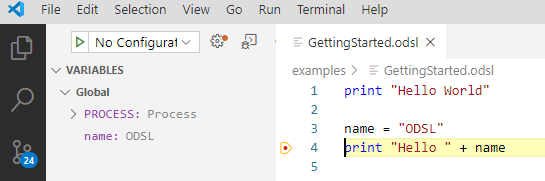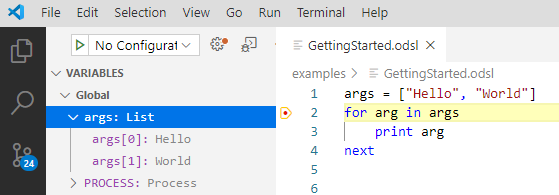# ODSL Code Basics

QuickStart Module

This quickstart module gives you an introduction to variables, looping, conditional statements and testing.

## Hello World​

Every self-respecting tutorial must start with a simple hello world program - so here it is:

``print "Hello World"``

This simple one-liner will print the text Hello World to the output console.

## Variables​

ODSL is untyped, this means you don’t need to declare the type of the variable when creating it.

The easiest way to create a variable is to assign a value to it:

``name = "ODSL"print "Hello " + name``

In the above code example, we have created a variable called name and assigned the string “ODSL” to it. We then use that variable in our print statement to produce the output string Hello ODSL.

info

A Note on Debugging in VS Code

Debugging allows you to step through code checking to see what happens to the variables as the code is run.

In VSCode, if you put a breakpoint in the above code on the line to print “Hello “ + name, we can inspect the name variable as shown below:You can see there are 2 variables in the Global scope:

• PROCESS
• name

You can see the name variable with the value ODSL

Variable scopes are covered in a later section

The PROCESS variable will be covered later on when we run workflows, processes and scripts

### Scalar variables​

The scalar class of variable encompasses the following types:

• String
• Number (both integer and decimal numbers)
• Boolean (true/false)

To create a scalar variable, you simply assign a value to it, e.g.

``MyString = "OpenDataDSL"MyNumber = 12.33MyBoolean = true``

### Objects​

Objects are a general dynamic variable that can have other variables added to it as properties. You create an object using the Object() constructor, then you assign properties to it, e.g.

``MyObject = Object()MyObject.value = 22MyObject.description = "My Dynamic Object"``

### Lists​

Lists are arrays of other variables that you would generally use to iterate through. You can create a list either using the List() constructor or using an array initialiser, here are some examples:

``MyList=List()MyList1=[]MyList2=["this", "is", "my", "list", 1, 2, 3]``

As you can see, you don't need to add the same type of variable in the list.

### Adding more items to the list​

To add more items to the list, use the add method on the list, e.g.

``MyList2.add("test")print MyList2``

This outputs:

``[thisismylist123test]``

## Other variable types​

A deep dive into all the other variable types is in the next module of this track.

## Control Statements​

Most modern standard programming languages have some programming control statements in order to control the execution flow and ODSL is no different, so let’s explore them.

### Looping and iterating​

You can loop through a sequence of numbers or iterate though a list of variables using a for statement, so imagine we simply want to loop through 10 numbers and print them to the output console, this is achieved as follows:

``for i = 1 to 10  print inext``

This code will print out the numbers 1 to 10 in the output console

You can also iterate through a list or array or variables using a for statement:

``args = ["Hello", "World"]for arg in args    print argnext``

Notice how we construct an array using square brackets ‘[' and ']’.

See how this looks in the debugger:### Condition statements​

#### If this is true?​

A condition statement routes the code execution according to the condition outcome and an if statement is used to check this.

Extending our looping example, we can add a condition to change the output:

``for i = 1 to 10    if i > 5        print i    endnext``

Now the output will only show the number 6 to 10

We can also check for multiple conditions using the elseif command:

``for i = 1 to 10    if i > 5        print i    elseif i == 3        print "Three"    endnext``

This will now output the word “Three” when i is equal to 3

Notice the check for equality that uses ==, this distinguishes the check from an assignment which is a single = sign

We can also add a ‘catch-all’ else statement for any other values not falling in the other conditions:

``for i = 1 to 10    if i > 5        print i    elseif i == 3        print "Three"    else        print "Something else"    endnext``

The output from this snippet of code is:

``Something elseSomething elseThreeSomething elseSomething else678910``

Note that the else statement has to be the last part of the if condition

#### While this is true​

Another conditional control statement is the while command, this combines the looping of a for statement with the conditional flow of an if statement.

``x=5y=10while x < y    x = x + 1    print xend``

In this code snippet, we initialise 2 variables x and y with the values 5 and 10 respectively.

We then enter the while loop testing the condition that x is less than y.

The statements in the loop will execute once and the condition is tested again.

This continues until the condition is false.

danger

Beware that the while control statement can go into an endless loop: e.g. the following code doesn’t change the value of x, so it will never be less than y

``x=5  y=10  while x < y      print x  end``

``// This is an arrayargs = ["Hello", "World"] // A comment on the same line``

``/*    We need to loop through the    array and print it out*/for arg in args    print argnext``

Comments are ignored when the program is run

## Testing​

Repeatable testing is an important part of any developers toolkit, which is why we have added some specific functions that you can add into your code.

Testing generally requires assertions, these effectively say 'assert that this is correct'.

For example:

``MyVariable = 23.22assertEquals(23.22, MyVariable)``

The assertEquals function has 2 parameters:

• An expected value
• An actual test value

If they exactly match, then the test passes, if it doesn't match, your script will halt running and you will receive a message something like:

`` 11:0 Assertion Error: Actual does not match expected -     Expected: 23.230000    Actual: 23.220000``

### Assertion functions​

Below is the full list of test assertion functions:

FunctionDescriptionSyntaxExample
assertEqualsTests for equalityassertEquals(expected, actual)assertEquals("test",myval)
assertTrueTests a conditionassertTrue(condition)assertTrue(myval == "test")
assertFalseTests a conditionassertFalse(condition)assertFalse(a<5)
assertTypeTests a variable is a certain typeassertType(var,"type")assertType(myval,"Scalar")
assertNullTests to see if a variable or property is nullassertNull(var)assertNull(test)
assertHasPropertyTests to see if a variable has a non-null propertyassertHasProperty(var,"name")assertHasProperty(myval,"name")